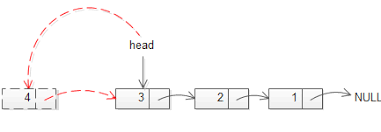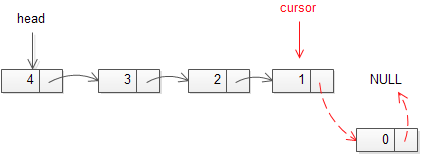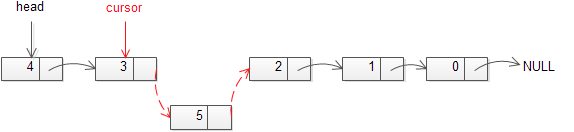You can insert/add elements to either the beginning, middle or end of the linked list.

### insert a node at  beginning:

*Allocate memory for new node

*Store data

*Change next of new node to point to head

*Change head to point to recently created new node

for example, if the given linked list is  17->25->40->45 and we add item 12  at the front ,then the linked list becomes 12->17->25->40->45Below is the 4 step process of adding a new node at the front of Linked List declared at the beginning of this post:

c++:

```void push(struct Node** head_ref, int new_data)
{
/* 1. allocate node */
Node* new_node = new Node;

/* 2. store data  */
new_node->data  = new_data;

/* 3. Make next of new node as head */

/* 4. move the head to point to the new node */
}```

Java:

```public void push(int new_data)
{
/* 1 & 2: Allocate the Node &
Put in the data*/
Node new_node = new Node(new_data);

/* 3. Make next of new Node as head */

/* 4. Move the head to point to new Node */
}  ```

The time complexity of inserting a node at start of the List is O(1).

### Inserting a Node at the End:

*Allocate memory for new node
*Store data
*Traverse to last node
*Change next of last node to recently created nodebelow is the proceess of adding a new node at the end of linked list:
c++:
``` void append(struct Node** head_ref, int new_data)
{
/* 1. allocate node */
Node* new_node = new Node;

struct Node *cursor= *head_ref;  /* used in step 5*/

/* 2. put in the data  */
new_node->data  = new_data;

/* 3. This new node is going to be the last node,
so make next of it as NULL*/
new_node->next = NULL;

/* 4. If the Linked List is empty, then make
the new node as head */
{
return;
}

/* 5. Else traverse till the last node */
while (cursor->next != NULL)
cursor = cursor->next;

/* 6. Change the next of cursor node */
cursor->next = new_node;
return;
} ```
java:
```public void append(int new_data)
{
/* 1. Allocate the Node &
2. Put in the data
3. Set next as null */
Node new_node = new Node(new_data);

/* 4. If the Linked List is empty, then make the
{
return;
}

/* 4. This new node is going to be the last node, so
make next of it as null */
new_node.next = null;

/* 5. Else traverse till the last node */
while (last.next != null)
last = last.next;

/* 6. Change the next of last node */
last.next = new_node;
return;
} ```

The time complexity of this operation is O(N) where N is the number of nodes in the Linked List as one have to traverse the complete list in order to find the last node.

### inserting a node after a given node:

Inserting a Node after a given Node is also similar to the above process.
*One have to first allocate the new Node
*change the next pointer of the newly created node to the next of the previous node
*the next pointer of the previous node to point to the newly created node.(the cursor node is nothing but the previous node)

below is the proceess of adding a new node after a given node of linked list:
c++:

``` void insertAfter(struct Node* prev_node, int new_data)
{
/* 1. check if the given prev_node is NULL */
if (prev_node == NULL)
{
printf("the given previous node cannot be NULL");
return;
}

/* 2. allocate new node */
Node* new_node = new Node;

/* 3. put in the data  */
new_node->data  = new_data;

/* 4. Make next of new node as next of prev_node */
new_node->next = prev_node->next;

/* 5. move the next of prev_node as new_node */
prev_node->next = new_node;
}    ```

Java:
```public void insertAfter(Node prev_node, int new_data)
{
/* 1. Check if the given Node is null */
if (prev_node == null)
{
System.out.println("The given previous node cannot be null");
return;
}

/* 2. Allocate the Node &
3. Put in the data*/
Node new_node = new Node(new_data);

/* 4. Make next of new Node as next of prev_node */
new_node.next = prev_node.next;

/* 5. make next of prev_node as new_node */
prev_node.next = new_node;
} ```

The time complexity of inserting a node at start of the List is O(1).

if we dont know the previous node we have to traverse to node just before the required position of new node Change next pointers to include new node in between

we can do it like this:
c++:
```struct node *cursor = head;

for(int i=1; i < position; i++) {
if(cursor->next != NULL) {
cursor = cursor->next;
}
}```

darkmode